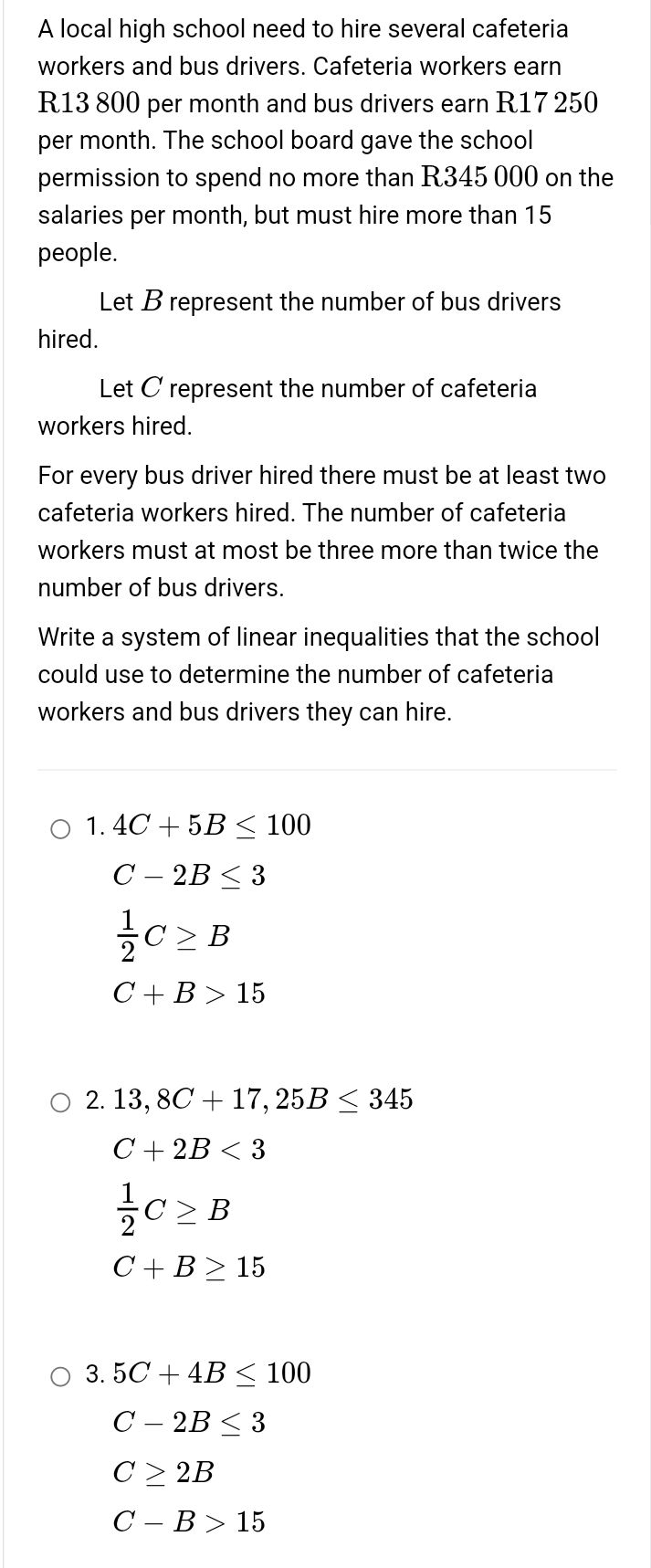### Still have math questions?

Algebra
QuestionA local high school need to hire several cafeteria workers and bus drivers. Cafeteria workers earn R13 $$800$$ per month and bus drivers earn R17 $$250$$ per month. The school board gave the school permission to spend no more than R345 $$000$$ on the salaries per month, but must hire more than $$15$$ people. Let $$B$$ represent the number of bus drivers hired. Let $$C$$ represent the number of cafeteria workers hired. For every bus driver hired there must be at least two cafeteria workers hired. The number of cafeteria workers must at most be three more than twice the number of bus drivers. Write a system of linear inequalities that the school could use to determine the number of cafeteria workers and bus drivers they can hire.

1. $$4 C + 5 B \leq 100$$

$$C - 2 B \leq 3$$

$$\frac { 1 } { 2 } C \geq B$$

$$C + B > 15$$

2. $$13,8 C + 17,25 B \leq 345$$

$$C + 2 B < 3$$

$$\frac { 1 } { 2 } C \geq B$$

$$C + B \geq 15$$

3. $$5 C + 4 B \leq 100$$

$$C - 2 B \leq 3$$

$$C \geq 2 B$$

$$C - B > 15$$